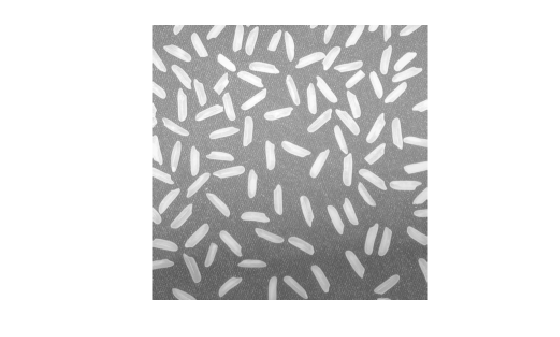Description

example

Z = imadd(X,Y) adds each element in array X with the corresponding element in array Y and returns the sum in the corresponding element of the output array Z.

Examples

collapse all

This example shows how to add two uint8 arrays with truncation for values that exceed 255.

X = uint8([ 255 0 75; 44 225 100]);
Y = uint8([ 50 50 50; 50 50 50 ]);
Z = 2x3 uint8 matrix

255    50   125
94   255   150

Read two grayscale uint8 images into the workspace.

Add the images. Specify the output as type uint16 to avoid truncating the result.

Display the result.

imshow(K,[])Read an image into the workspace.

Add a constant to the image.

Display the original image and the result.

imshow(I)figure
imshow(J)Input Arguments

collapse all

First array, specified as a numeric array or logical array of any dimension.

Second array to be added to X, specified as a numeric or logical array of the same size and class as X, or a numeric scalar of type double.

Output Arguments

collapse all

Sum, returned as a numeric array of the same size as X. Z is the same class as X unless X is logical, in which case Z is data type double. If X is an integer array, elements of the output that exceed the range of the integer type are truncated, and fractional values are rounded.